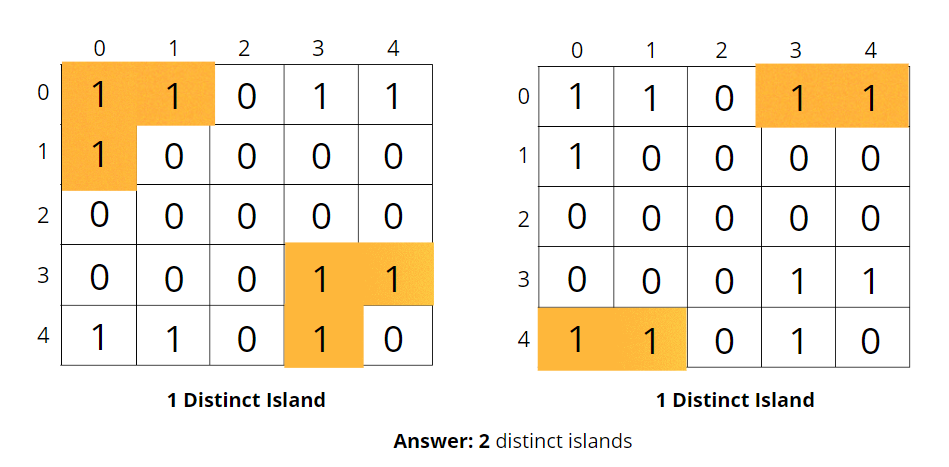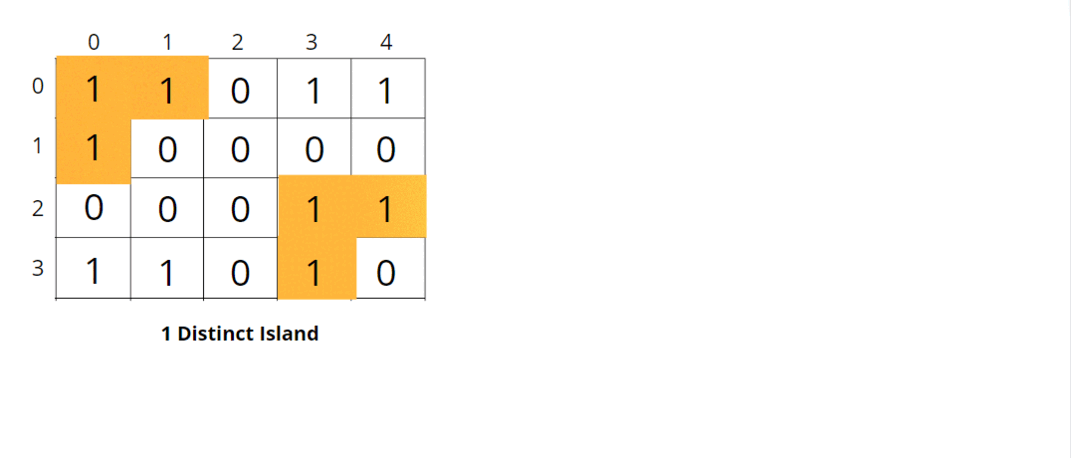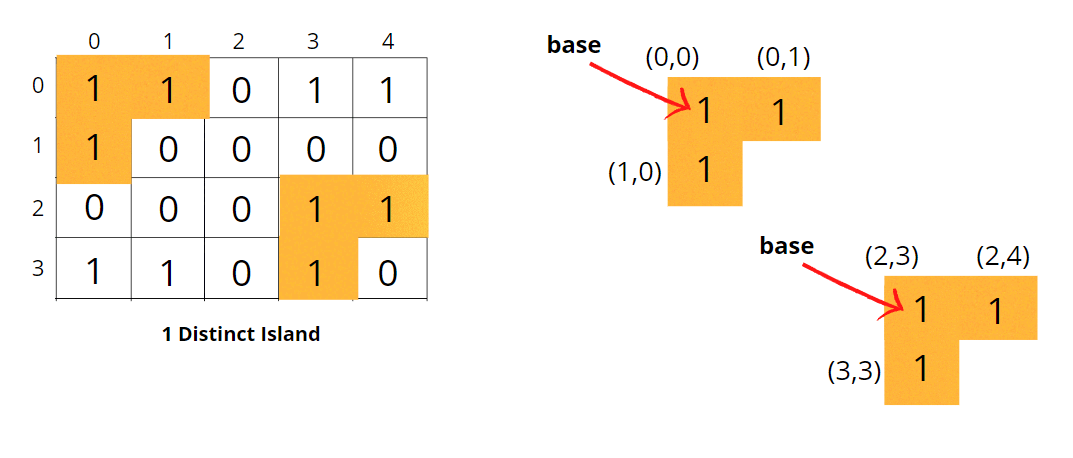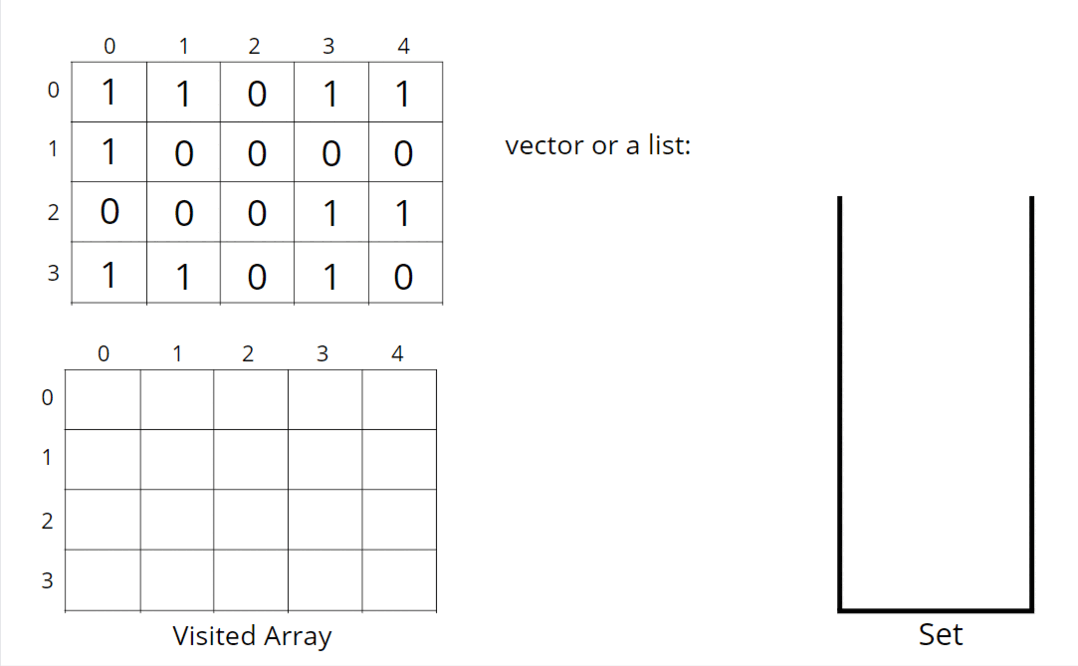# Number of Distinct Islands

Problem Statement: Given a boolean 2D matrix grid of size N x M. You have to find the number of distinct islands where a group of connected 1s (horizontally or vertically) forms an island. Two islands are considered to be distinct if and only if one island is equal to another (not rotated or reflected).

Examples:

Example 1:

Input:

Output: 1

Explanation: Island at the top left corner is the same as the island at the bottom right corner.

Example 2:

Input:

Output: 3

Explanation: Island at the top right corner is the same as the island at the bottom left corner.

### Solution

Disclaimer: Don’t jump directly to the solution, try it out yourself first.

### Intuition:.

Consider the following example, the two islands in the first figure might look identical but they are rotated so you can’t say they are the same, hence 2 distinct islands; whereas in the second figure, both islands are the same so only 1 distinct island; resulting in overall 3 distinct islands.Consider another example, the two islands in the first figure and another two islands in the second figure are the same, hence total of 2 distinct islands.Depending on the shape of the island formed, we count the number of islands.

The question arises how to store these shapes?

We can store the shapes in a set data structure, then the set will return unique islands. We can store the coordinates in a vector or a list.But how to figure out if the coordinates stored in the set data structure are identical? We can call one of the starting points a base, and subtract the base coordinates from the land’s coordinates (Cell Coordinates – Base coordinates). Now the list will be similar as illustrated.NOTE: Make sure to follow a particular traversal and a particular order pattern, so that list ordering remains the same for every cell.

How do store distinct islands?

We’ve done this type of problem on a number of islands. This is an expansion of the number of islands so refer to that article for the same.

### Approach:

We can follow either of the traversal techniques. We will be solving it using DFS traversal, but you can apply BFS traversal as well.

DFS is a traversal technique that involves the idea of recursion and backtracking. DFS goes in-depth, i.e., traverses all nodes by going ahead, and when there are no further nodes to traverse in the current path, then it backtracks on the same path and traverses other unvisited nodes.

The algorithm steps are as follows:

• For DFS traversal to start and visit all the nodes connected to itl, we need a start node and a visited array. Create a corresponding visited array, which will be an NxM matrix.
• We will be looping through the NxM cell, and for every unvisited node in the visited matrix, we will call the DFS for it, so that the DFS now expands and marks all the cells attached to it as visited.
• DFS function calls will run through all the unvisited neighboring land cells in the 4 directions.
• At the start of the DFS, we mark the nodes as visited and stored, and then we check for their adjacent nodes. If they are unvisited, we call DFS for them one by one.
• During the DFS call, every time we visit a cell, we will store its resultant coordinates into a list, and when the overall DFS gets completed, we will store the list into a set DS.
• Keep repeating these steps for every unvisited cell and visit the entire island.
• The set data structure contains the list of unique islands only, so return the length of the set. In this way, we will get the number of distinct islands.

Consider the following illustration to understand how DFS traverses the grid and stores the distinct islands. (only 2 islands are traversed in the illustration)How do set boundaries for 4 directions?

The 4 neighbors will have the following indexes:Now, either we can apply 4 conditions or follow the following method. From the above image, it is clear that the delta change in the row is -1, +0, +1, +0. Similarly, the delta change in the column is 0, +1, +0, -1.  So we can apply the same logic to find the neighbors of a particular pixel (<row, column>).

Code:

## C++ Code

``````#include<bits/stdc++.h>

using namespace std;

class Solution {
private:
void dfs(int row, int col, vector < vector < int >> & vis,
vector < vector < int >> & grid, vector < pair < int, int >> & vec, int row0,
int col0) {
// mark the cell as visited
vis[row][col] = 1;

// coordinates - base coordinates
vec.push_back({
row - row0,
col - col0
});
int n = grid.size();
int m = grid.size();

// delta row and delta column
int delrow[] = {-1, 0, +1, 0};
int delcol[] = {0, -1, 0, +1};

// traverse all 4 neighbours
for (int i = 0; i < 4; i++) {
int nrow = row + delrow[i];
int ncol = col + delcol[i];
// check for valid unvisited land neighbour coordinates
if (nrow >= 0 && nrow < n && ncol >= 0 && ncol < m &&
!vis[nrow][ncol] && grid[nrow][ncol] == 1) {
dfs(nrow, ncol, vis, grid, vec, row0, col0);
}
}
}
public:
int countDistinctIslands(vector < vector < int >> & grid) {
int n = grid.size();
int m = grid.size();
vector < vector < int >> vis(n, vector < int > (m, 0));
set < vector < pair < int, int >>> st;

// traverse the grid
for (int i = 0; i < n; i++) {
for (int j = 0; j < m; j++) {
// if not visited and is a land cell
if (!vis[i][j] && grid[i][j] == 1) {
vector < pair < int, int >> vec;
dfs(i, j, vis, grid, vec, i, j);
// store in set
st.insert(vec);
}
}
}
return st.size();
}
};

int main() {

vector<vector<int>> grid{
{1, 1, 0, 1, 1},
{1, 0, 0, 0, 0},
{0, 0, 0, 0, 1},
{1, 1, 0, 1, 1}};

Solution obj;
cout << obj.countDistinctIslands(grid) << endl;

}``````

Output:  3

Time Complexity: O(N x M x log(N x M)) + O(NxMx4) ~ O(N x M), For the worst case, assuming all the pieces as land, the DFS function will be called for (N x M) nodes, and for every node, we are traversing for 4 neighbors, it will take O(N x M x 4) time. Set at max will store the complete grid, so it takes log(N x M) time.

Space Complexity ~ O(N x M), O(N x M) for the visited array and set takes up N x M locations at max.

## Java Code

``````import java.util.*;

class Solution {
private void dfs(int row, int col, int[][] vis,
int[][] grid, ArrayList < String > vec, int row0, int col0) {
// mark the cell as visited
vis[row][col] = 1;

// coordinates - base coordinates
vec.add(toString(row - row0, col - col0));
int n = grid.length;
int m = grid.length;

// delta row and delta column
int delrow[] = {-1, 0, +1, 0};
int delcol[] = {0, -1, 0, +1};

// traverse all 4 neighbours
for (int i = 0; i < 4; i++) {
int nrow = row + delrow[i];
int ncol = col + delcol[i];
// check for valid unvisited land neighbour coordinates
if (nrow >= 0 && nrow < n && ncol >= 0 && ncol < m &&
vis[nrow][ncol] == 0 && grid[nrow][ncol] == 1) {
dfs(nrow, ncol, vis, grid, vec, row0, col0);
}
}
}
private String toString(int r, int c) {
return Integer.toString(r) + " " + Integer.toString(c);
}

int countDistinctIslands(int[][] grid) {
int n = grid.length;
int m = grid.length;
int vis[][] = new int[n][m];
HashSet < ArrayList < String >> st = new HashSet < > ();

// traverse the grid
for (int i = 0; i < n; i++) {
for (int j = 0; j < m; j++) {
// if not visited and is a land cell
if (vis[i][j] == 0 && grid[i][j] == 1) {
ArrayList < String > vec = new ArrayList < > ();
dfs(i, j, vis, grid, vec, i, j);
// store it in HashSet
}
}
}
return st.size();
}

public static void main(String[] args) {
int grid[][] = {
{1, 1, 0, 1, 1},
{1, 0, 0, 0, 0},
{0, 0, 0, 0, 1},
{1, 1, 0, 1, 1}};

Solution ob = new Solution();
int ans = ob.countDistinctIslands(grid);
System.out.println(ans);
}
}

class Pair {
int first;
int second;
public Pair(int first, int second) {
this.first = first;
this.second = second;
}
}``````

Output:  3

Time Complexity: O(N x M x log(N x M)) + O(NxMx4) ~ O(N x M), For the worst case, assuming all the pieces as land, the DFS function will be called for (N x M) nodes, and for every node, we are traversing for 4 neighbors, it will take O(N x M x 4) time. Set at max will store the complete grid, so it takes log(N x M) time.

Space Complexity ~ O(N x M), O(N x M) for the visited array and set takes up N x M locations at max.

Special thanks to Vanshika Singh Gour for contributing to this article on takeUforward. If you also wish to share your knowledge with the takeUforward fam, please check out this articleIf you want to suggest any improvement/correction in this article please mail us at [email protected]